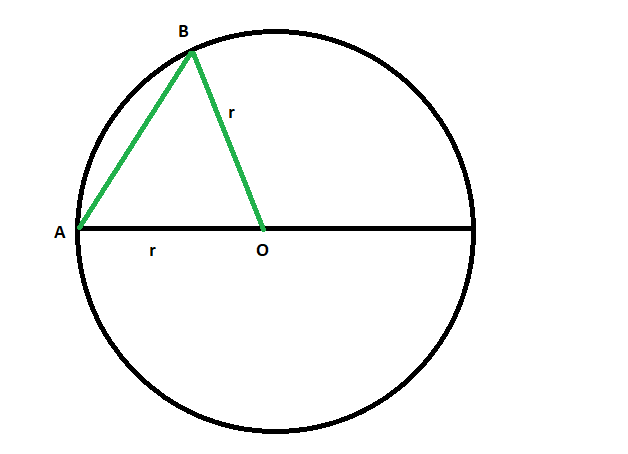Open in App
Not now

# Find the Diameter or Longest chord of a Circle

• Last Updated : 07 Jun, 2022

Given a circle with radius ‘r’ is given, the task is to find the diameter or longest chord of the circle.
Examples:

```Input: r = 4
Output: 8

Input: r = 9
Output: 18```Proof that the Longest chord of a circle is its Diameter:

• Draw circle O and any chord AB on it.
• From one endpoint of the chord, say A, draw a line segment through the centre. That is, draw a diameter.
• Now draw a radius from centre O to B.
• By the triangle inequality,

```AB < AO + OB
= r + r
= 2r
= d```
• So, any chord that is not a diameter will be smaller than a diameter.
• So the largest chord is a diameter

Approach:

• The Longest chord of any circle is its diameter.
• Therefore, the diameter of a circle is twice the radius of it.

`Length of the longest chord or diameter = 2r`
•

Below is the implementation of the above approach:

## C++

 `// C++ program to find` `// the longest chord or diameter` `// of the circle whose radius is given`   `#include ` `using` `namespace` `std;`   `// Function to find the longest chord` `void` `diameter(``double` `r)` `{` `    ``cout << ``"The length of the longest chord"` `         ``<< ``" or diameter of the circle is "` `         ``<< 2 * r << endl;` `}`   `// Driver code` `int` `main()` `{`   `    ``// Get the radius` `    ``double` `r = 4;`   `    ``// Find the diameter` `    ``diameter(r);`   `    ``return` `0;` `}`

## Java

 `// Java program to find` `// the longest chord or diameter` `// of the circle whose radius is given` `class` `GFG ` `{` `    `  `// Function to find the longest chord` `static` `void` `diameter(``double` `r)` `{` `    ``System.out.println(``"The length of the longest chord"` `        ``+ ``" or diameter of the circle is "` `        ``+ ``2` `* r);` `}`   `// Driver code` `public` `static` `void` `main(String[] args)` `{` `    `  `    ``// Get the radius` `    ``double` `r = ``4``;`   `    ``// Find the diameter` `    ``diameter(r);` `}` `}`   `// This code contributed by Rajput-Ji`

## Python3

 `# Python3 program to find` `# the longest chord or diameter` `# of the circle whose radius is given`   `# Function to find the longest chord` `def` `diameter(r):`   `    ``print``(``"The length of the longest chord"` `        ``,``" or diameter of the circle is "` `        ``,``2` `*` `r)`     `# Driver code`   `# Get the radius` `r ``=` `4`   `# Find the diameter` `diameter(r)`   `# This code is contributed by mohit kumar`

## C#

 `// C# program to find` `// the longest chord or diameter` `// of the circle whose radius is given` `using` `System;`   `class` `GFG ` `{` `    `  `// Function to find the longest chord` `static` `void` `diameter(``double` `r)` `{` `    ``Console.WriteLine(``"The length of the longest chord"` `        ``+ ``" or diameter of the circle is "` `        ``+ 2 * r);` `}`   `// Driver code` `public` `static` `void` `Main(String[] args)` `{` `    `  `    ``// Get the radius` `    ``double` `r = 4;`   `    ``// Find the diameter` `    ``diameter(r);` `}` `}`   `// This code has been contributed by 29AjayKumar`

## PHP

 ``

## Javascript

 ``

Output:

`The length of the longest chord or diameter of the circle is 8`

Time Complexity: O(1)

Auxiliary Space: O(1)

My Personal Notes arrow_drop_up
Related Articles